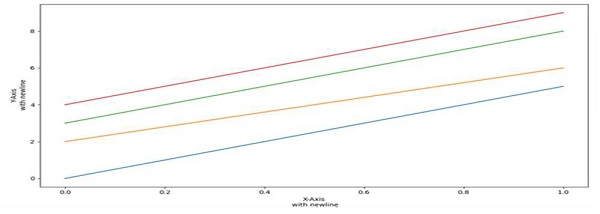# Putting a newline in Matplotlib label with TeX in Python

PythonMatplotlibServer Side ProgrammingProgramming

#### Beyond Basic Programming - Intermediate Python

Most Popular

36 Lectures 3 hours

#### Practical Machine Learning using Python

Best Seller

91 Lectures 23.5 hours

#### Practical Data Science using Python

22 Lectures 6 hours

The following program code shows how you can plot a newline in matplotlib label with Tex.

## Steps

• Setup X-axis and Y-axis labels for the diagram with \n to plot a newline in the labels.

• Set the current .rcParams for axes facecolor; the group is axed.

• Use a cycler to set the color for the group of lines. The color list consists of ‘r’ for red, ‘g’ for green, ‘b’ for blue, and ‘y’ for yellow.

• The cycler class helps to create a new Cycler object from a single positional argument, a pair of positional arguments, or the combination of keyword arguments.

• Plot the number of lines with different colors.

• Use plt.show() to show the figure.

## Example

import matplotlib.pyplot as plt
from cycler import cycler

plt.ylabel("Y-axis \n with newline")
plt.xlabel("X-axis \n with newline")

plt.rc('axes', prop_cycle=(cycler('color', ['r', 'g', 'b', 'y'])))

plt.plot([0, 5])
plt.plot([2, 6])
plt.plot([3, 8])
plt.plot([4, 9])
plt.show()

## Output